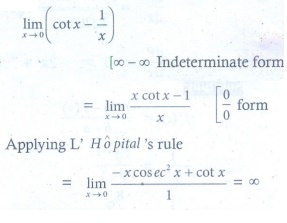# Chapter 7: Applications of Differential Calculus - Online Test

Q1.

The volume of a sphere is increasing in volume at the rate of 3π cm3 / sec .

The rate of change of its radius when radius is 1/2 cm
Explaination / Solution: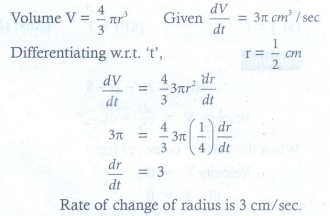Q2. A balloon rises straight up at 10 m/s. An observer is 40 m away from the spot where the balloon left the ground. The rate of change of the balloon’s angle of elevation in radian per second when the balloon is 30 metres above the ground.
Explaination / Solution: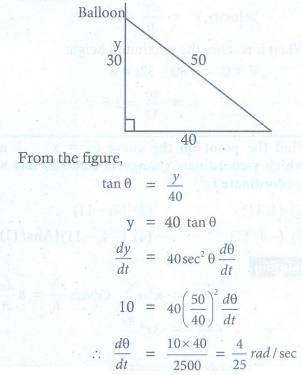Q3. The position of a particle moving along a horizontal line of any time t is given by s (t) = 3t2 − 2t − 8 . The time at which the particle is at rest is
Explaination / Solution: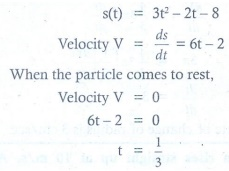Q4. A stone is thrown up vertically. The height it reaches at time t seconds is given by x = 80t −16t2 . The stone reaches the maximum height in time t seconds is given by
Explaination / Solution: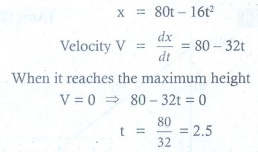Q5. The point on the curve 6y = x3 + 2 at which y-coordinate changes 8 times as fast as x-coordinate is
Explaination / Solution: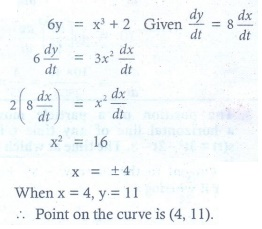Q6. The abscissa of the point on the curve f (x) = √(8−2x) at which the slope of the tangent is −0.25 ?
Explaination / Solution: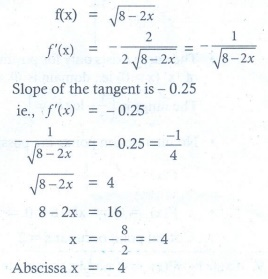Q7. The slope of the line normal to the curve f (x) = 2 cos 4x at x = π/12 is
Explaination / Solution: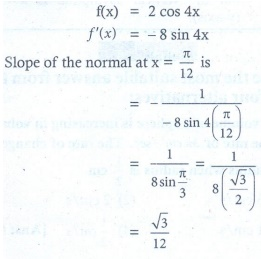Q8. The tangent to the curve y2 − xy + 9 = 0 is vertical when
Explaination / Solution: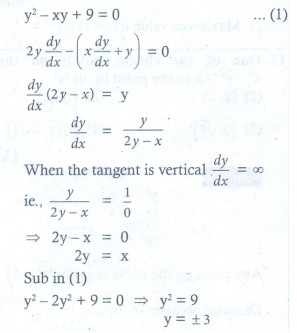Q9. Angle between y2 = x and x2 = y at the origin is
Explaination / Solution: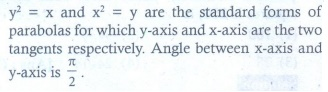Q10. The value of the limit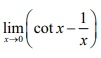is
Explaination / Solution: